# Logical Reasoning - Online Test

Q1. Find the minimum number of straight lines required to make the given figure.Explaination / Solution:

The figure may be labelled as shown.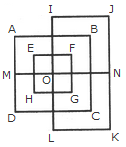The horizontal lines are IJ, AB, EF, MN, HG, DC and LK i.e. 7 in number.

The vertical lines are AD, EH, IL, FG, BC and JK i.e. 6 in number.

Thus, there are 7 + 6 = 13 straight lines in the figure.

Q2. Per capita income of slum dwellers is significantly lower than those living in better housing facilities.
Explaination / Solution:

Consider the following line of the passage: “Slum dwellers are characterized by low productivity and susceptible to poor health conditions.” Therefore, it can be assumed that the inference is true. Hence, option A is correct.

Q3. Find the number of triangles in the given figure.Explaination / Solution:

The figure may be labelled as shown.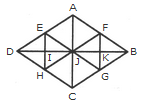The simplest triangles are AFJ, FJK, FKB, BKG, JKG, JGC, HJC, HIJ, DIH, DEI, EIJ and AEJ i.e. 12 in number.

The triangles composed of two components each are JFB, FBG, BJG, JFG, DEJ, EJH, DJH and DEH i.e. 8 in number.

The triangles composed of three components each are AJB, JBC, DJC and ADJ i.e. 4 in number.

The triangles composed of six components each are DAB, ABC, BCD and ADC i.e. 4 in number.

Thus, there are 12 + 8 + 4 + 4 = 28 triangles in the figure.

Q4. Majority of the slums in cities and towns in India are on prime private properties.
Explaination / Solution:

Consider the following lines of the passage: “In its most ambitious bid ever to house 6-crore slum dwellers and realize the vision of a slum free India, the government is rolling out a massive plan to build 50 lakh dwelling units in five years across 400 towns and cities. The program could free up thousands of acres of valuable government land across the country and generate crores worth of business for real estate developers.” Thus, the inference probably true. Hence, option B is correct.

Q5. Find the number of triangles in the given figure.Explaination / Solution:

The figure may be labelled as shown.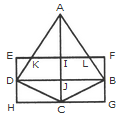The simplest triangles are AKI, AIL, EKD, LFB, DJC, BJC, DHC and BCG i.e. 8 in number.

The triangles composed of two components each are AKL, ADJ, AJB and DBC i.e. 4 in number.

The triangles composed of the three components each are ADC and ABC i.e. 2 in number.

There is only one triangle i.e. ADB composed of four components.

Thus, there are 8+ 4 + 2 + 1= 15 triangles in the figure.

Q6. Direction: Below is given a passage followed by several possible inferences which can be drawn from the facts stated in the passage. You have to examine each inference separately in the context of the passage and decide upon its degree of truth or falsity.
Development of land occupied by slums in cities of India will not have any effect on the common public.
Explaination / Solution:

Consider the last line of the passage: “The government believes that better housing facilities will address social issues and also have a multiplier effect and serve as an economic stimulus.” The inference probably false. Hence, option E is correct.

Q7. Find the number of triangles in the given figure.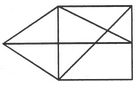Explaination / Solution:

The figure may be labelled as shown.The simplest triangles are ABF, BIC, CIH, GIH, FGE and AFE i.e. 6 in number.

The triangles composed of two components each are ABE, AGE, BHF, BCH, CGH and BIE i.e. 6 in number.

The triangles composed of three components each are ABH, BCE and CDE i.e. 3 in number.

Hence, the total number of triangles in the figure = 6 + 6 + 3 = 15.

Q8. Directions: Read the given statements carefully and answer the questions which follow.
After the government announced a compensatory policy for farmers in return for the acquisition of their land, the farmers demanded a written proof of the policy. Which of the following could possibly be a cause for demanding the written proof ?
Explaination / Solution:

The farmers demand a certain percentage of share out of the revenue generated by the government off their lands.

Q9. Find the number of triangles in the given figure.Explaination / Solution:

The figure may be labelled as shown.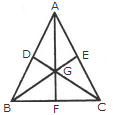The simplest triangles are AGE, EGC, GFC, BGF, DGB and ADG i.e. 6 in number.

The triangles composed of two components each are AGC, BGC and ABG i.e. 3 in number.

The triangles composed of three components each are AFC, BEC, BDC, ABF, ABE and DAC i.e. 6 in number.

There is only one triangle i.e. ABC composed of six components.

Thus, there are 6 + 3 + 6 + 1 = 16 triangles in the given figure.

Q10. Directions: Read the given statements carefully and answer the questions which follow.
In a governmental initiative, which started a couple of years ago, school teachers of a particular State were engaged in teaching the illiterate every evening after the regular school hours.

Which of the following can be an effect of the statement given above ?
Explaination / Solution:

The literacy rate in the State has improved significantly this year.